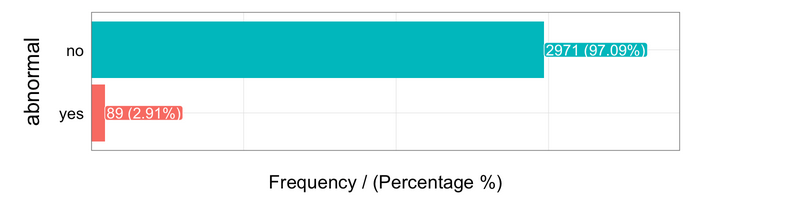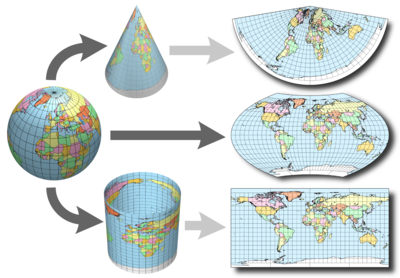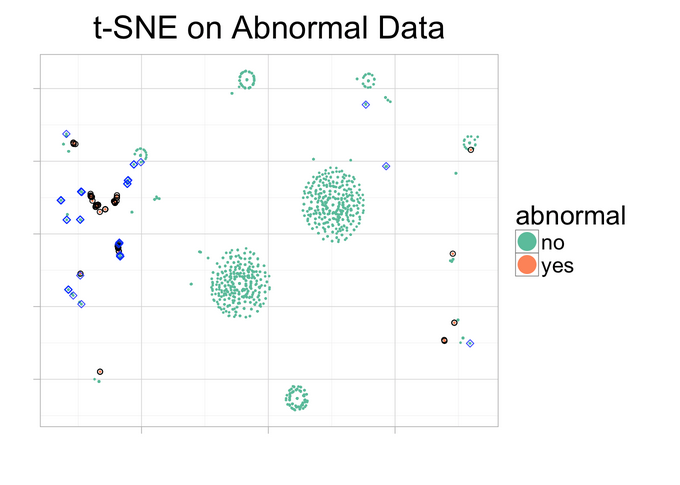Discover and enable the integrations you need to solve identityAuth0 Marketplace
Machine Learning

# Machine Learning for Everyone - Part 2: Spotting anomalous data

Case study in R reviewing common concepts regarding how to validate, run and visualize a predictive model on production ranking the most suspicious cases.

January 27, 2017Machine Learning

# Machine Learning for Everyone - Part 2: Spotting anomalous data

Case study in R reviewing common concepts regarding how to validate, run and visualize a predictive model on production ranking the most suspicious cases.

January 27, 2017

### Overview

We're going to analyze data that contain cases flagged as abnormal. So we'll build a predictive model in order to spot cases that are not currently flagged as abnormal, but behaving like ones that are.

Topics are:

• Creating a predictive model (random forest)
• Introduction to ROC value (model performance)
• Understanding of label and score prediction
• Inspection of suspicious cases (audit)
• Prediction and dimension reduction (t-SNE)

### Let's start!

This post contains R code and some machine learning explanations, which can be extrapolated to other languages such as Python. The idea is to create a case study giving the reader the opportunity to recreate results. You will need the following:

Note: There are some points oversimplified in the analysis, but hopefully you'll become curious to learn more about this topic, in case you've never done a project like this.

First, install and load the packages (libraries) containing the functions we'll use in this project, and load the data.

``````# delete these installation lines after 1st run
install.packages("caret")
install.packages("funModeling")
install.packages("Rtsne")``````
``````library(caret)
library(funModeling)
library(Rtsne)

## download data from github, if you have any problem go directly to github: https://github.com/auth0/machine-learning-post-2-abnormal-behavior
url_git_data = "https://raw.github.com/auth0/machine-learning-post-2-abnormal-behavior/master/data_abnormal.txt"

The data contains the following columns:

``colnames(data)``
``````##   "id"       "abnormal" "var_1"    "var_2"    "var_3"    "var_4"
##   "var_5"    "var_6"    "var_7"    "var_8"``````

We are going to predict column `abnormal` based on `var_1`, `var_2` ... `var_8` variables.

Inspecting target variable:

``freq(data, "abnormal")``Almost 3 percent of cases are flagged as abnormal.

Next, we create the predictive model using Random Forest, doing the model parameter tuning with `caret` library using 4-fold cross-validation optimized for the ROC metric. We'll come back to this later.

You can find the basics of random forest in the first post of this series.

``````################################################
## MODEL CREATION:
################################################
set.seed(999)
## Setting the validation metric: Cross-Validation 4-fold.
fitControl = trainControl(method = "cv",
number = 4,
classProbs = TRUE,
summaryFunction = twoClassSummary)

## Creating the model, given the cross-validation method.
fit_model = train(abnormal ~ var_1 + var_2 + var_3 + var_4 + var_5 + var_6 + var_7 + var_8,
data = data,
method = "rf",
trControl = fitControl,
verbose = FALSE,
metric = "ROC")``````

There are some important things to note about the last layout:

The `mtry` column indicates a parameter that is optimized by the library `caret`.

The selection is based on the ROC metric. This metric goes from 0.5 to 1 and indicates how well the model distiguishes between `True Positve` and `False Positive` rates. The higher the better.

### Choosing the "best" model

Predictive models have some parameters that can be tuned in order to improve predictions based on the input data. In the last example, `caret` chooses the best model configuration based on a desired accuracy metric---ROC, in this case.

Random forest does not have many parameters to tune compared with other similar gradient boosting machines. The parameter to tune was `mtry`.

`caret` tested 3 different values of `mtry`, and the value which maximizes the ROC value is `6`.

Cross-validating results is really important; you can get more information in ref. 

### What is the ROC value?

This is a long -long- topic, but here we try to introduce you to some aspects to start becoming familiar with it.

#### Some history

During World War II, radar detected if an airplane was coming or not, so they sent out the following alerts:

• `Hey, a plane was spotted!` (positive prediction)
• `Be easy, no plane is coming....` (negative prediction)

These outcomes can be `True` or `False`, so we have four possibilites:

1- If the detection of `Hey, a plane was spotted!` is `True`, it is called a `True Positive`.

2- On the other hand, if `Hey, a plane was spotted!` is `False`, the result is a `False Positive`, which implies that the radar (predictive model) said a plane was coming but there isn't actually a plane out there.

3- The radar says `Be easy, no plane is coming....` and the real result is Positive: It's a `True Negative`.

4- The radar says `Be easy, no plane is coming....` and the real result is Negative: It's a `False Negative`.

#### Abnormal example data

In the data we used to build the model, we have:

• `Positive` is when abnormal=`yes`.
• `Negative` is when abnormal=`no`.

Normally we associate the positive value with the less representative value, which is the one we are trying to explain and the least common.

#### Analysis of possibilites

Points 1 and 3 imply the radar (or the predictive model) asserts the prediction.

With points 2 and 4, the model failed---it predicted one thing and it was actually the opposite.

The ROC value measures the trade-off between the `True Positive` and `True Negative` rates. This is because we need to be sure about what the model is saying when it detects a `Positive` outcome:

Is this `Positive` prediction reliable or not?

#### Usage in medicine

This accuracy metric is so powerful that it is used in other fields, such as medicine, to measure the accuracy of certain diagnoses:

"The flu test for this patient was positive": if this result is confirmed after a while or a second test, we've got a true positve.

It is used in many tests in which the result is either `true` or `false`; it's very important to know if we can trust this result.

#### Usage in Auth0

We did some proof of concept to automatically spot the most suspicious login cases in order to boost current anomaly detection feature, and ROC curve was a good option to test the predictive model sensitivity.

#### Understanding the extremes

Knowing extreme ROC values is a good approach to better understanding it:

• An ROC value of `1` indicates all the `Positive` values returned by the model are correct. Perfect model.
• An ROC value of `0.5` indicates all the `Positive` values returned by the model have results similar to random guessing. This is the worst model.

See more about ROC in ref. 

### Going back to the predictive model

We talked about the predictive model output, which is something like: `positive / negative` or `yes / no`. But it's better to work with probabilities (also known as score) so the output of any predictive model (in binary or multi-label class prediction) should be the score.

For a longer explanation, see ref. .

Once we get the score value, we can select all cases above a threshold and label them as `abnormal`.

We assign the probability of being `abnormal=yes` to each case:

``data\$score=predict(fit_model\$finalModel, type="prob")[,2]``

We can kept with those cases with are actually `abnormal=no`:

``````# filtering cases...
data_no_abnormal=subset(data, abnormal=="no")``````

We obtain the top 2% of cases with the highest score for being abnormal:

``````# obtaining the score to filter top 2%
cutoff=quantile(data_no_abnormal\$score, probs = c(0.98))

# filtering most suspicious cases
data_to_inspect=subset(data_no_abnormal, score>cutoff)``````

And here we've got in `data_to_inspect` ~ 60 cases which are actually no abnormal, but they are showing a behavior of being suspicious.

Displaying only the first 6 suspicious IDs to further inspect...

``head(data_to_inspect\$id)``
``##   59  94 105 107 224 259``

Is there a way to visualize how the predictive model "sees" data and assigns the probabilities?

We've built a machine learning model in order to know the abnormal cases, using random forest.

Predictive models handle several variables at the same time, which is different from the classical reporting approach, in which you see two or three variables in one plot.

In the following example, you'll see how eight variables are mapped in only two dimensions. In this case there are eight, but it could be thousands. This is quite fancy since it is like a compression of the information.

You've probably already seen this "complex" technique: in geographical maps. They map three dimensions into two:There are several ways to do this with data; the most popular is probably Principal Component Analysis -aka PCA- (however, this doesn't lead to good visualizations). The one we used in this post is t-Distributed Stochastic Neighbor Embedding, which is also implemented in languages other than R. More info at ref. .

Google did a live demo based on text data, see ref. .

#### Hands on R!

First some data preparation--excluding id and score variables to create the t-SNE model. Also, converting all string variables into numeric ones since t-SNE doesn't support this type of variable (one-hot encoding or placeholder variables).

Actually, we're going to map 17 variables (not eight) because of this transformation.

``````# excluding id column and score variable
data_2=data[,!(names(data) %in% c("id", "score"))]
d_dummy = dummyVars(" ~ .", data = data_2)
data_tsne = data.frame(predict(d_dummy, newdata = data))``````

Now we create the t-SNE model, adding it to the `score` variable we created before.

``````set.seed(999)
tsne_model = Rtsne(as.matrix(data_tsne), check_duplicates=FALSE, pca=TRUE, perplexity=30, theta=0.5, dims=2)
d_tsne = as.data.frame(tsne_model\$Y)
d_tsne\$abnormal = as.factor(data\$abnormal)
d_tsne\$score=data\$score
d_tsne = d_tsne[order(d_tsne\$abnormal),]``````

Now the magic! Plotting the resulting t-SNE model which maps 17 variables in two dimensions :)

``````ggplot(d_tsne, aes(x=V1, y=V2, color=abnormal)) +
geom_point(size=0.25) +
guides(colour=guide_legend(override.aes=list(size=6))) +
xlab("") + ylab("") +
ggtitle("t-SNE on Abnormal Data") +
theme_light(base_size=20) +
theme(axis.text.x=element_blank(),
axis.text.y=element_blank()) +
geom_point(data=d_tsne[d_tsne\$abnormal=="yes",], color="black", alpha=1,shape=21) +
geom_point(data=d_tsne[d_tsne\$abnormal=="no" & d_tsne\$score>=cutoff,], color="blue", alpha=0.8,shape=5, aes(color=Class)) +
scale_colour_brewer(palette = "Set2")``````### But what are we seeing there? Analysis

• Blue rhomboids represent the cases which are actually not flagged as abnormal, but are flagged as "highly abnormal" by the predictive model (this is what was analyzed before).

• Pink points represent the cases which are actually flagged as abnormal.

• Green points are the cases which are not actually abnormal, having a low likelihood of being one of them.

Similar cases tend to be closer in the plot.

Pink cases are close to the blue ones. These are the cases predicted as highly suspicious and that share similar behavior with the ones actually flagged as abnormal.

That's why we left the `score` variable out of the t-SNE model creation. This model put closely together some cases that were actually `abnormal` and `not abrnormal`, and this makes sense since these cases were flagged by the random forest as suspicious ones.

Look at the biggest islands made of green points---there are no abnormal points, flagged or predicted, near them.

#### Conclusions

The cases flagged as abnormal, plus the top 2 percent of suspicious ones detected by the random forest, are mapped closer together away from the normal cases, because they behave differently. This is one way of uncovering the information in the data.

Time to play with your own data :)